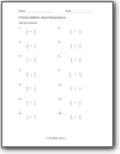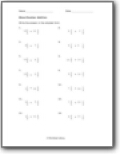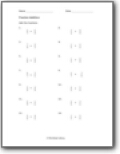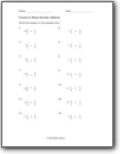There are whole numbers, and there are fractions in mathematics. All numbers starting from 0 are whole numbers. Fractions, on the other hand, are the portion of a whole number. There are two types of fractions: proper fractions and improper fractions. Proper fractions are the ones where the denominator is greater than the numerator. The value of these fractions lies between 0 and 1. The improper fractions are those where the denominator is less than the numerator. The value of these fractions is greater than 1. Be it a proper or improper fraction, you can similarly add them. The right way to add fractions is by making the denominators the same. This can be done by finding out the LCM of the denominators. Once you have found the LCM, you can multiply both the numerator and the denominator with the multiple that would provide the desired results. For example, you are dealing with two fractions; a/b and c/d. The LCM here will be bd. For this, you need to multiply the first fractions with d. It will then become ad/bd. The second fraction has to be multiplied by b. It will become bc/bd. Once you have the same denominators, you can add the numerators and turn them into a single fraction.###### Same Denominators

Add these values that all share the same bottom number.Write the answer in the simplest form.###### Mixed Denominators

There are both like and unlike denominators in these sums.###### Adding Fractions & Mixed Numbers

One of the values is mixed numbers and the other value is a straight up fraction.